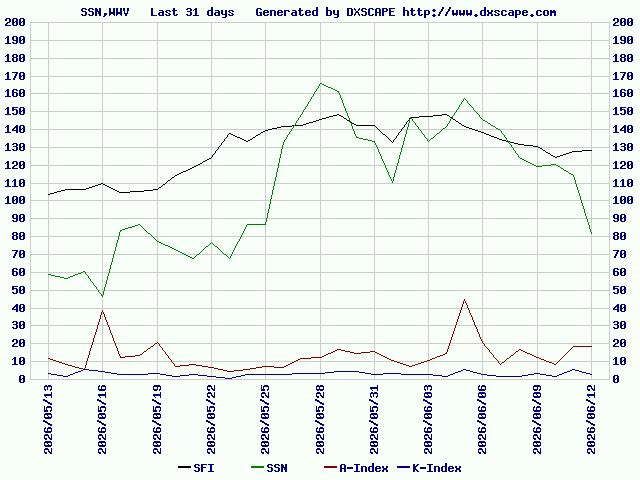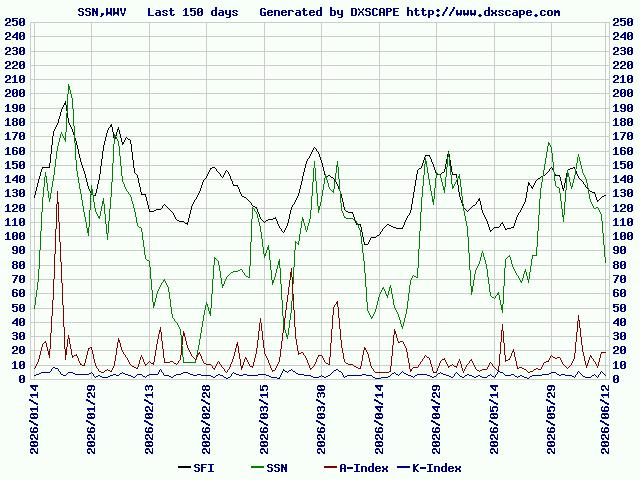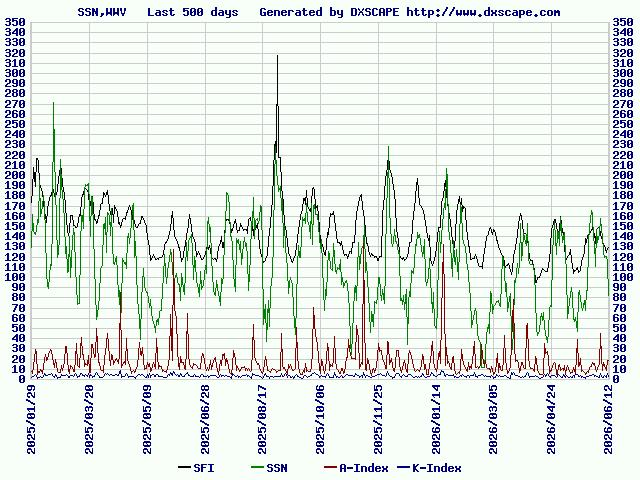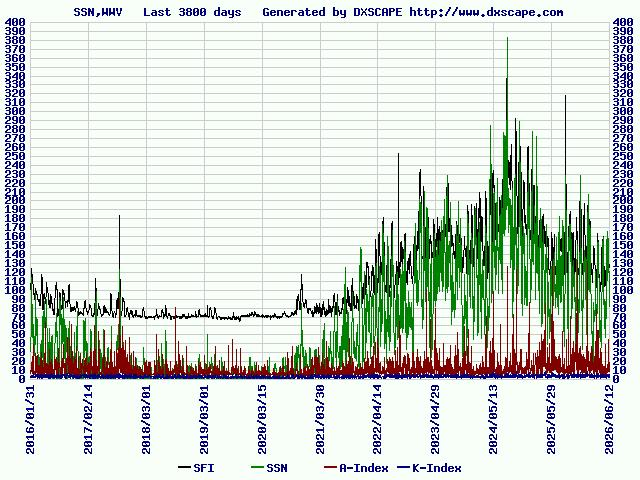Solar activity
Sun Spot Number , WWV plot

Updated every 0010Z

```0309Z = 1209JST   SFI=158 SSN=146 A=9 K=2
``````From Wikipedia

The A-index provides a daily average level for geomagnetic activity.
Because of the non-linear relationship of the K-scale to magnetometer
fluctuations, it is not meaningful to take averages of a set of K indices.
What is done instead is to convert each K back into a linear scale
called the "equivalent three hourly range" a-index (note the lower case).
The daily A index is merely the average of eight "a" indices.

Thus, for example, if the K indices for the day were 3 4 6 5 3 2 2 1,
the daily A index is the average of the equivalent amplitudes:

A = (15 + 27 + 80 + 48 + 15 + 7 + 7 + 3)/8 = 25.25

```

References

753959